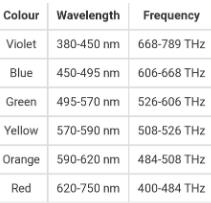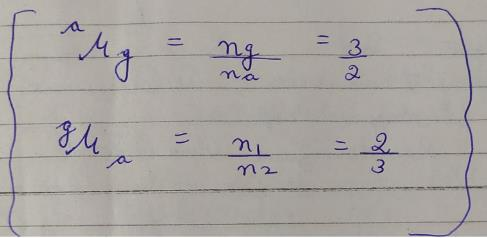Courses

# Test: Ray Optics - 2

## 25 Questions MCQ Test Science Class 10 | Test: Ray Optics - 2

Description
This mock test of Test: Ray Optics - 2 for UPSC helps you for every UPSC entrance exam. This contains 25 Multiple Choice Questions for UPSC Test: Ray Optics - 2 (mcq) to study with solutions a complete question bank. The solved questions answers in this Test: Ray Optics - 2 quiz give you a good mix of easy questions and tough questions. UPSC students definitely take this Test: Ray Optics - 2 exercise for a better result in the exam. You can find other Test: Ray Optics - 2 extra questions, long questions & short questions for UPSC on EduRev as well by searching above.
QUESTION: 1

### When white light passes through a prism, it splits into its component colours. This phenomenon is called

Solution:

The dispersion of light is the phenomenon of splitting of a beam of white light into its seven constituent colours when passed through a transparent medium. It was discovered by Isaac Newton in 1666. Newton discovered that light is made up of seven different colours. He passed a beam of sunlight through a glass prism. The glass prism split the light into a band of seven colours on his wall. He called this band of colours the ‘spectrum’. Thus the spectrum is a band of seven colours which is obtained by splitting of white light by a glass prism. The order of colours from the lower end of spectrum is violet (V), indigo (I), blue (B), green (G), yellow (Y), orange (O), and red (R). The sequence of the 7 colours so obtained in a spectrum can be remembered by using the acronym ‘VIBGYOR’.

QUESTION: 2

### Quantity which does not changes during refraction is

Solution:

Frequency of light does not change on refraction, when a ray of light passes from one medium to another, it's direction changes because of change in speed of light from one medium to another.

QUESTION: 3

### A deviation in the path of a ray of light can be produced

Solution:

The bending of light as it passes from one medium to another is called refraction. The angle and wavelength at which the light enters a substance and the density of that substance determine how much the light is refracted. The bending occurs because light travels more slowly in a denser medium.

Hence, by a glass prism as well as a rectangular glass slab

QUESTION: 4

If you want to see your full image then the, minimum size of the plane mirror

Solution:

In order to see full image of a person, the minimum size of the mirror should be one half the person's height. This is so because, in reflection, the angle of incidence is equal to angle of reflection.

QUESTION: 5

The wavelengths corresponding to violet, yellow and red lights are λv , λy and λr respectively.

Solution:

Explanation:- because we know, the wavelength of red colour is the maximum, then comes yellow, and then Violet.QUESTION: 6

An object A is placed at a distance d in front of a plane mirror. If one stands directly behind the object at a distance S from the mirror, then the distance of the image.of A from the individual is

Solution:

The image has the dimensions as the object and is the same distance behind the mirror as the object is in front of the mirror.
In the case, the object A is placed at a distance d in front of a plane mirror and the person stands right behind the object and the total distance between the person and the mirror is S. This is inclusive of the distance of the object from the mirror.
Therefore, the distance of the image from the individual to the image that is formed at a distance d on the other side is given as
S+d.

QUESTION: 7

A light bulb is placed between two plane mirrors inclined at an angle of 60°. The number of images formed are

Solution:

Let, the angle of inclination be Theta ° Since the formula is 360 / theta - 1
Mirrors are inclined at an angle of 60°
360/60 - 1 = 5
Therefore, the number of images formed is 5.

QUESTION: 8

A person of height 1.8 standing at the centre of a room having equal dimensions of 10 m wishes to see the full image of the back wall in the mirror fixed on the front wall. The minimum height of the plane mirror needed for this purpose is

Solution:

The minimum heights needed for this purpose is 10/3m.

QUESTION: 9

Two plane mirrors inclined at an angle to one another have an object placed between them. If five images of the object are observed, the maximum possible angle between the mirrors is

Solution:

Given, no. of images = 5
We know,
No. Of images = 360/ theta  - 1
=>  5 = 360/ theta - 1
=>  6 = 360/theta
=> theta = 360/6
=> angle = 60°

QUESTION: 10

In case of a thick plane mirror multiple images arc formed. The brightest of all the images will be

Solution:

When an object is observed through a thick mirror, silvered at its back, a large number of images are obtained. Out of all the images, the second image is the brightest. In this case, the first image is formed by reflection at the first surface, which is the unsilvered surface of the mirror. The second image is formed after reflection from rear silvered surface, which sends maximum amount of light. Rest of the images formed are due to internal multiple reflections which occur inside the mirror. These images contain lesser amount of light and so their intensity gradually decreases.

QUESTION: 11

Indicate the only correct statement.

Solution:
QUESTION: 12

The focal length of a concave mirror is f and the distance from the object to the principal focus is x. The magnification obtained will be

Solution:
1/f=1/p+1/q

1/f-1/p=1/q

1/f-1/x+f=1/q

x/f(x+f)=1/q

=>q=f(x+f)/x

Now MagnificatiOn i.e

Size of image/size of object=q/p

={f(x+f)/x}x(x+f)

=f(x+f)/x(x+f)

=f/x.
QUESTION: 13

In a museum a child walks towards a large concave mirror. He will see that

Solution:

Explanation:- Concave mirrors can form inverted and real images and also virtual and erect images. Size can be smaller, larger or of the same size depending on the position of the object. Smaller than the size of the object, always.

If the mirror is a convex mirror, your image (actual size) will be smaller than you are. If the mirror is a concave mirror, your image (actual size) will usually be bigger than you are, depending on your distance from the mirror.

QUESTION: 14

The magnification of an object placed 10 cm from a convex mirror of radius of curvature 20 cm will be

Solution:
0.5 is the magnification

it came because....
u= -10cm
f=10 cm
v=5cm {1/f=1/v+1/u}

magnification=-v/u
m=5/10
magnification=0.5
QUESTION: 15

A concave mirror of focal length 10 cm produces an image five times as large as the object. If the image is in front of the mirror, the distance of the object from the mirror will be

Solution:

Here, f = -10 cmcm
m = -5 ( image is 5 times larger than the object)
⇒ -v/ u = -5
⇒ v = 5u
By using mirror formula,
1/f  = 1/u + 1/v
⇒ 1/-10   =  1/u +1/5u
⇒ 1/-10  = 6/5u
⇒ 5u  = 6*(-10) cm
⇒ u =   -60/5 cm
⇒ u  =  -12 cm
So, the distance of object is 12 cm from the mirror.

QUESTION: 16

To form an image twice the size of the object, using a convex lens of focal length 20 cm, the object distance must be –

Solution:

Given, f = 20 cm, h1 = 2h0

⇒ h1/ho = m = 2

v / u = 2 => v = 2u

1/ v - 1/ u = 1/ f ( lens formula)

⇒  1/2u - 1/-u  = 1/ 20

⇒ 3/ 2u = 1/ 20

⇒ u = 30 cm

So, option ( c) is the correct answer.

QUESTION: 17

Refractive index of glass w.r.t. air is 3/2. What is the refractie index of air w.r.t glass ?

Solution:If  refractive index of glass w.r.t air is 3/2 , then  refractive index of air w. r. t glass will be it's reciprocal ie. ⅔ ..

QUESTION: 18

The mirage is formed due to

Solution:
QUESTION: 19

A glass prism has refractive index 1.5 and the refracting angle 90° If a ray falls on it at an angle of incidence of 30°, then the angle of emergence will be

Solution:

We have, ( 1.5-1) *90
=  0.5 *90 ⇒ 45°
Now, 45-30+90°
⇒  105°
Angle of emergence has inclination with second surface.
⇒ 90 - 105
⇒ -15
This shows that light ray will not emerge out of the prism.

QUESTION: 20

When monochromatic light passes from vacuum to a material medium and vice versa; which of the following characteristics of light beam does not change ?

Solution:

When the monochromatic light passes from vacuum to medium there is no difference in frequency. Because whichever medium light enters there is no change in its frequency change in medium leads to change in velocity.

QUESTION: 21

A piece of glass when immersed in a transparent solution of refractive index 1.48 becomes almost invisible. The refractive index of glass used is

Solution:

If the glass and the liquid are having same refractive index then glass won't be  visible as it refracts light at same angle as the liquid does so that is reason that a glass piece is not visible in the liquid.

QUESTION: 22

Which of the following conditions are necessary for total internal reflection to take place at the boundary of two optical media?

I. Light is passing from optically denser medium to optically rarer medium.
II. Light is passing from optically rarer medium to optically denser medium.
III. Angle of incidence is greater than the critical angle.
IV. Angle of incidence is less than the critical angle.

Solution:
QUESTION: 23

The speed of light in vacuum is 3.0 × 108 m/s. If the refractive index of a transparent liquid is 4/3, then the speed of light in the liquid is

Solution:

Refractive index of water :-
Speed of light in vacuum / speed of light in water
4/3 = (3*108) /x
x  = 2.25 * 108 m/s

QUESTION: 24

The refractive indices of water and glass are 4/3 and 3/2 respectively. The refractive index of water with respect to glass is

Solution:

Refractive index of water, nw =  4/3
Refractive index  of  glass, ng =  3/2
Refractive index of water w. r. t glass
ngw = 4/3 / 3/2   =>  8/9

QUESTION: 25

Which of the following can be used to form a virtual image of an object?
I. convex lens
II. concave lens
III. concave mirror

Solution: